# Current Electricity and Electric Circuits Current Electricity To

• Slides: 34Current Electricity and Electric Circuits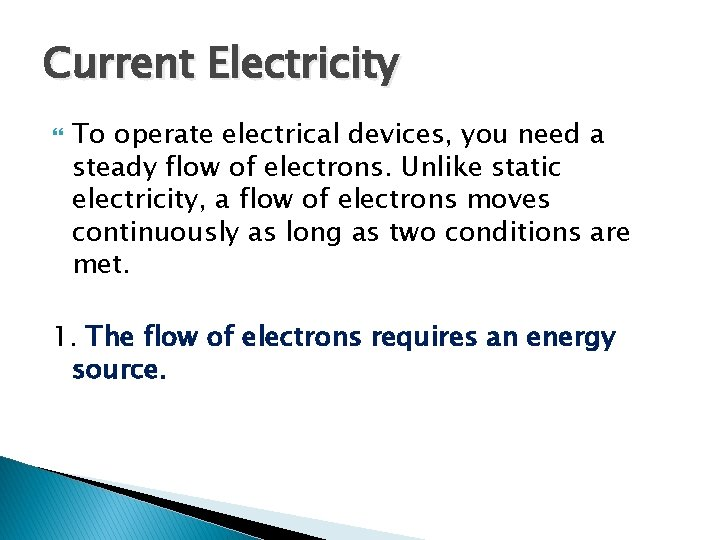Current Electricity To operate electrical devices, you need a steady flow of electrons. Unlike static electricity, a flow of electrons moves continuously as long as two conditions are met. 1. The flow of electrons requires an energy source.2. The electrons will not flow unless they have a complete path to flow through. ◦ This path is called an electrical circuit. The continuous flow of electrons in a circuit is called current electricity.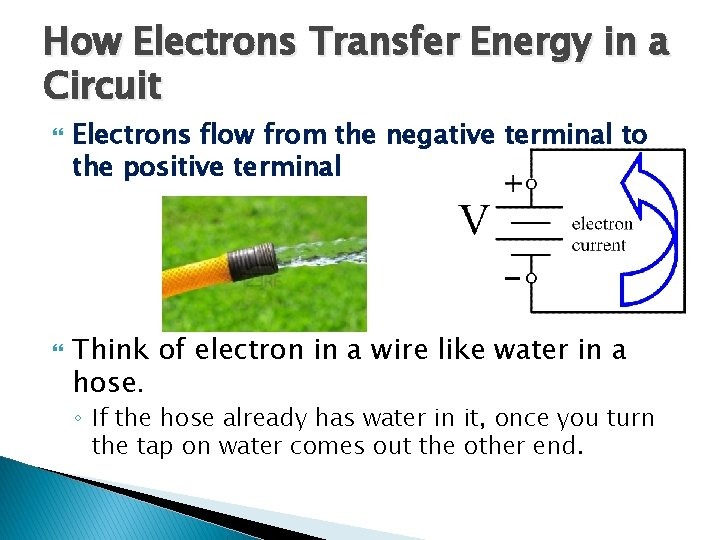How Electrons Transfer Energy in a Circuit Electrons flow from the negative terminal to the positive terminal Think of electron in a wire like water in a hose. ◦ If the hose already has water in it, once you turn the tap on water comes out the other end.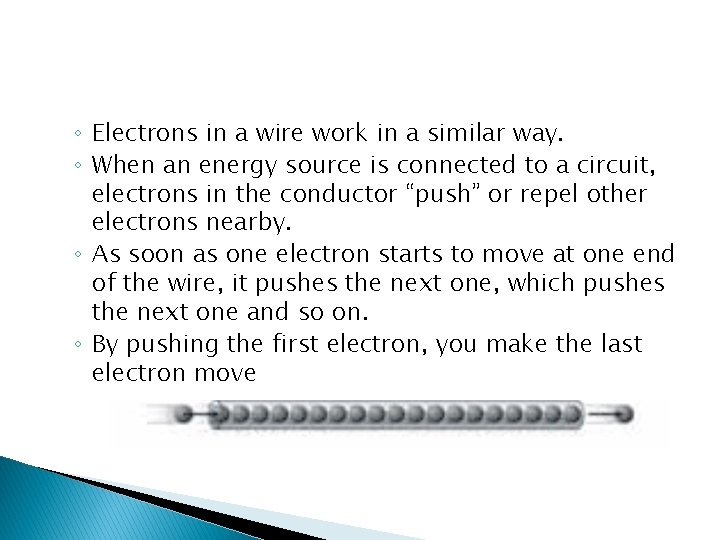◦ Electrons in a wire work in a similar way. ◦ When an energy source is connected to a circuit, electrons in the conductor “push” or repel other electrons nearby. ◦ As soon as one electron starts to move at one end of the wire, it pushes the next one, which pushes the next one and so on. ◦ By pushing the first electron, you make the last electron move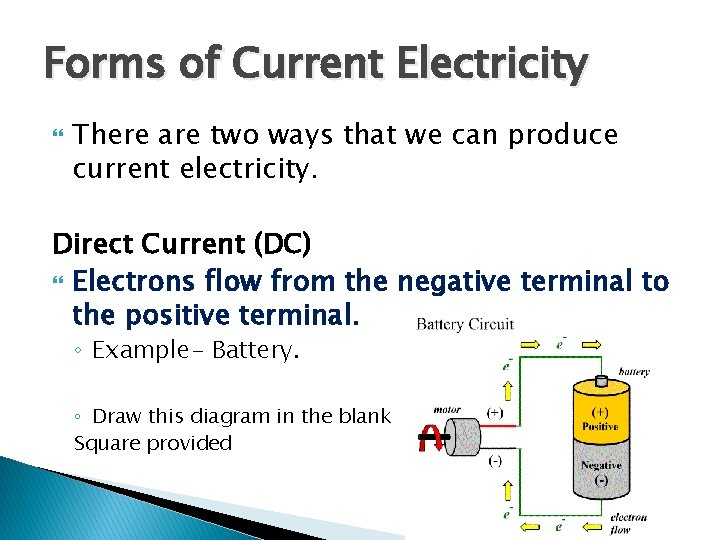Forms of Current Electricity There are two ways that we can produce current electricity. Direct Current (DC) Electrons flow from the negative terminal to the positive terminal. ◦ Example- Battery. ◦ Draw this diagram in the blank Square providedForms of Current Electricity Alternating Current (AC) Electrons move back and forth, alternating their direction many times every second. AC is used in more applications than DC as it is more efficient when traveling long distances. AC is used in all homes and work places.Electric Circuits A circuit includes: 1. Energy source – battery or generator 2. Conductor – allows the current to move through the circuit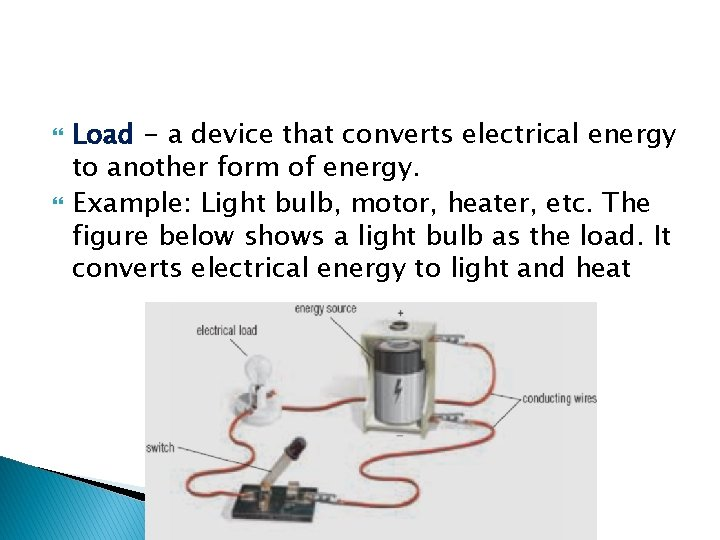Load - a device that converts electrical energy to another form of energy. Example: Light bulb, motor, heater, etc. The figure below shows a light bulb as the load. It converts electrical energy to light and heat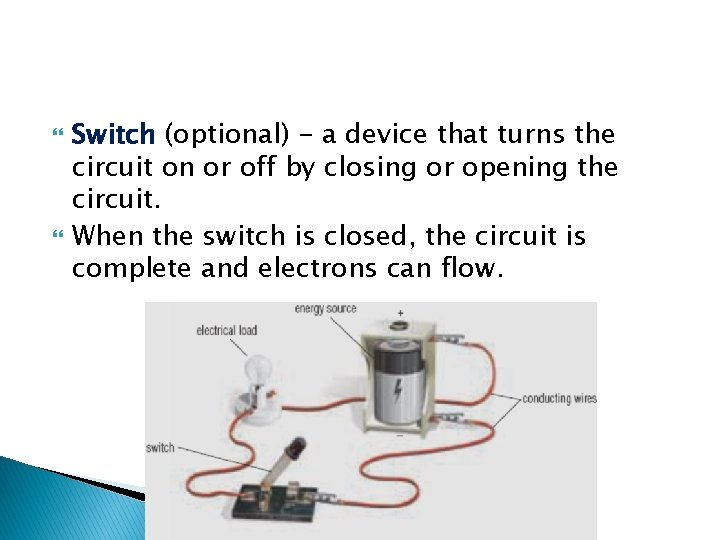Switch (optional) - a device that turns the circuit on or off by closing or opening the circuit. When the switch is closed, the circuit is complete and electrons can flow.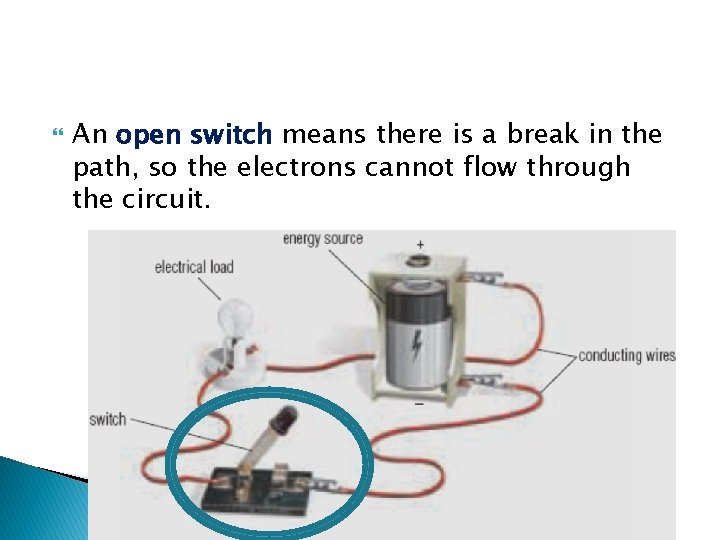An open switch means there is a break in the path, so the electrons cannot flow through the circuit.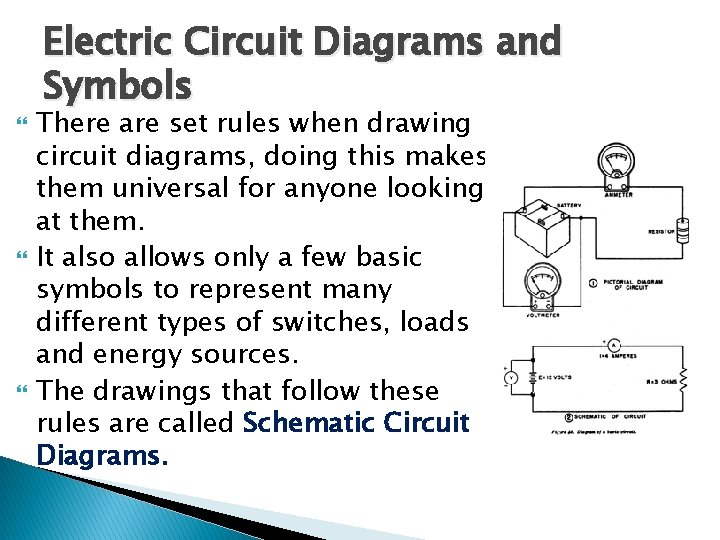Electric Circuit Diagrams and Symbols There are set rules when drawing circuit diagrams, doing this makes them universal for anyone looking at them. It also allows only a few basic symbols to represent many different types of switches, loads and energy sources. The drawings that follow these rules are called Schematic Circuit Diagrams.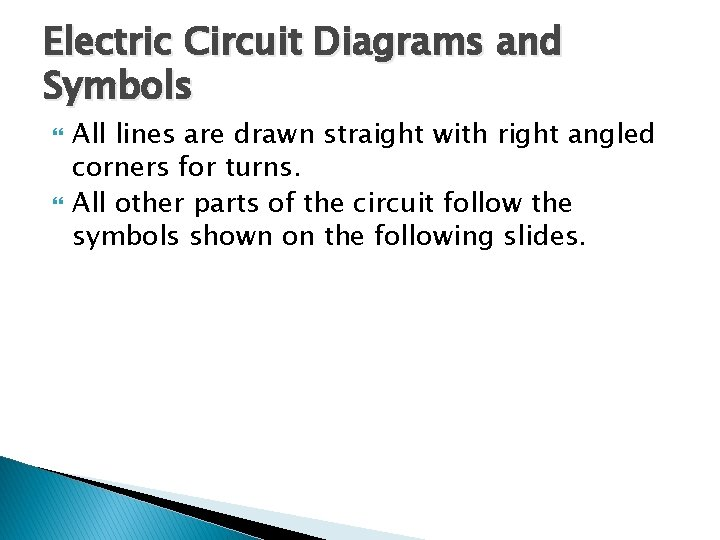Electric Circuit Diagrams and Symbols All lines are drawn straight with right angled corners for turns. All other parts of the circuit follow the symbols shown on the following slides.Conductor or wire Passes current easily from one part of a circuit to another.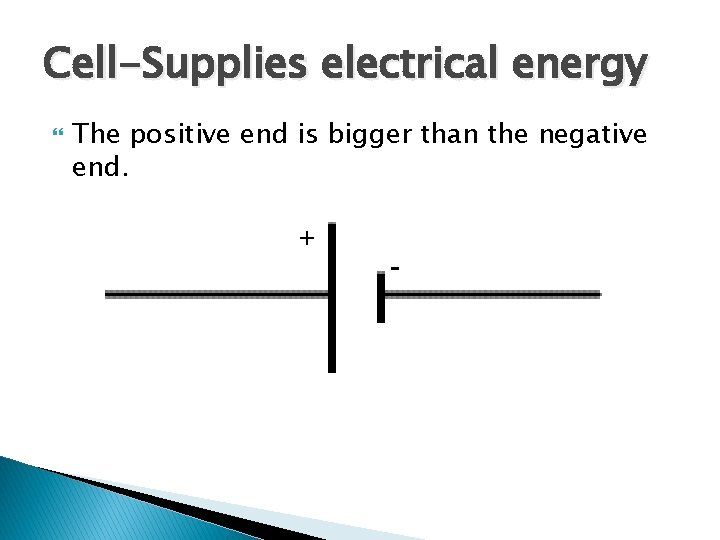Cell-Supplies electrical energy The positive end is bigger than the negative end. + -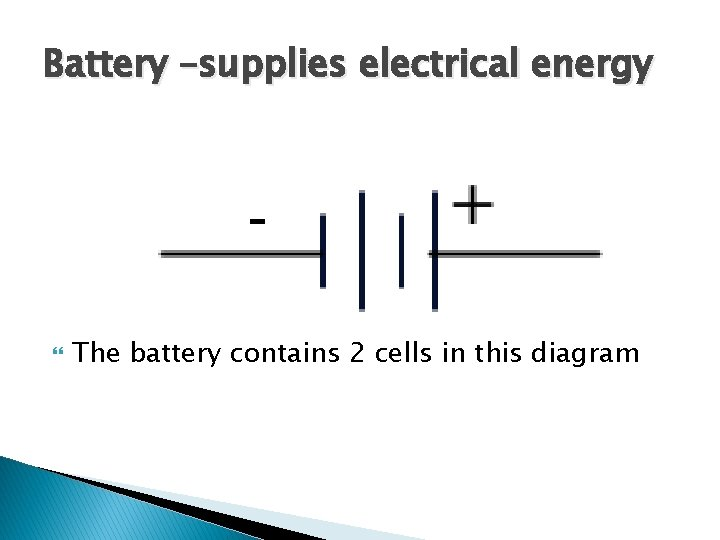Battery –supplies electrical energy The battery contains 2 cells in this diagramDC Source- Electrical energy source DC = Direct current, always flows one way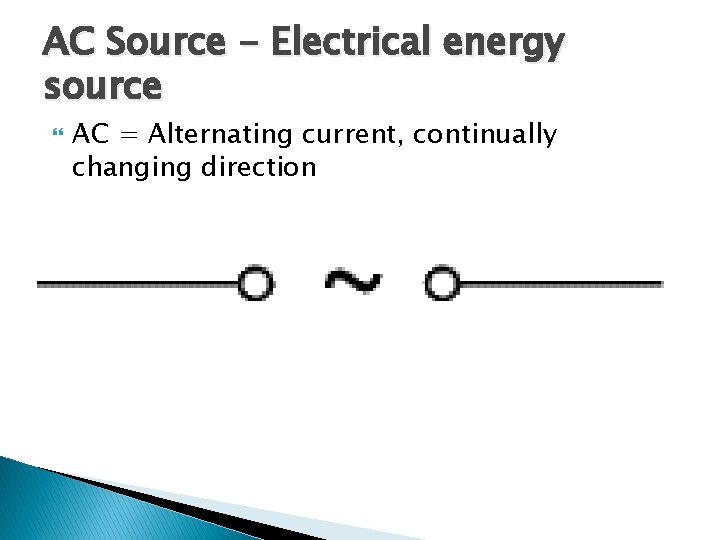AC Source - Electrical energy source AC = Alternating current, continually changing directionGround A connection to earthSwitch An on-off switch allows current to flow only when it is in the closed (on) position Open Closed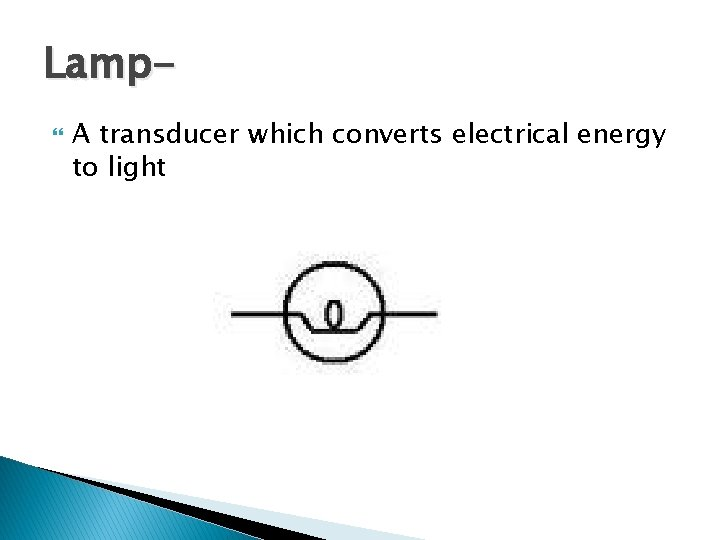Lamp A transducer which converts electrical energy to lightResistor A resistor restricts the flow of current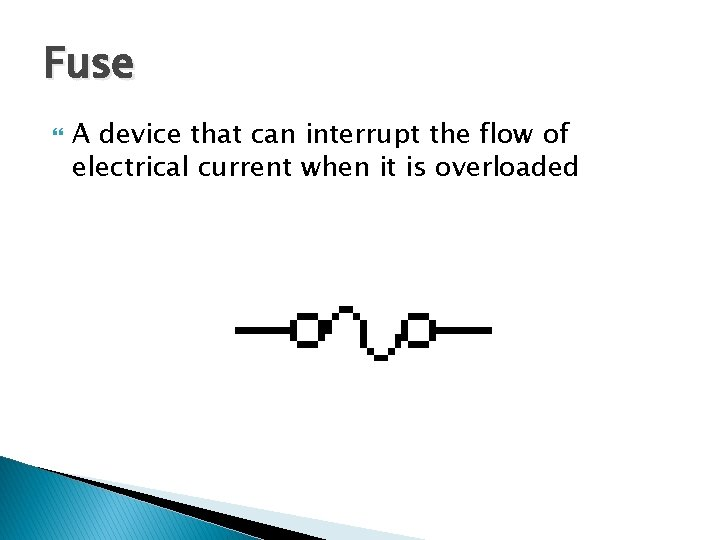Fuse A device that can interrupt the flow of electrical current when it is overloaded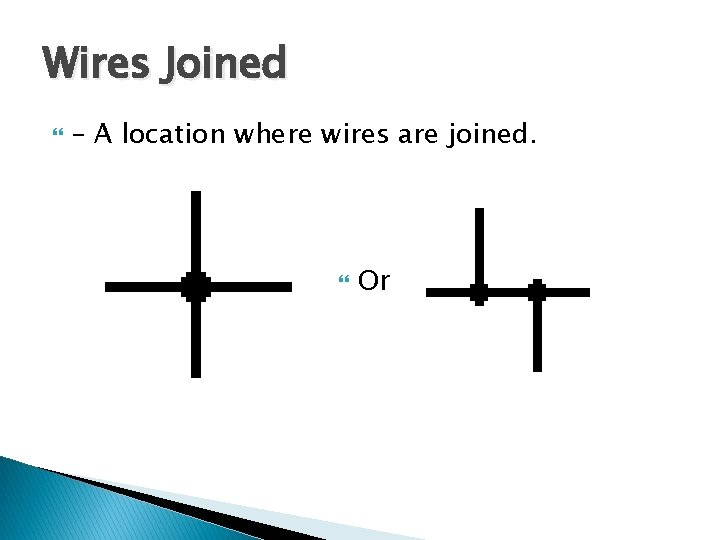Wires Joined – A location where wires are joined. Or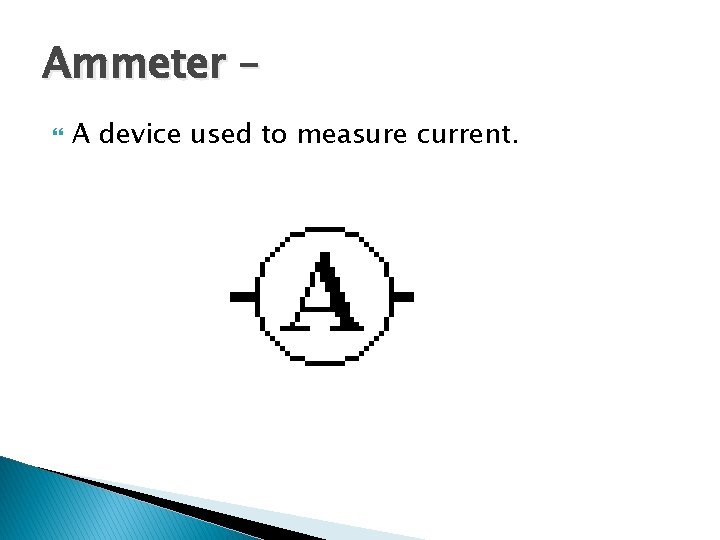Ammeter – A device used to measure current.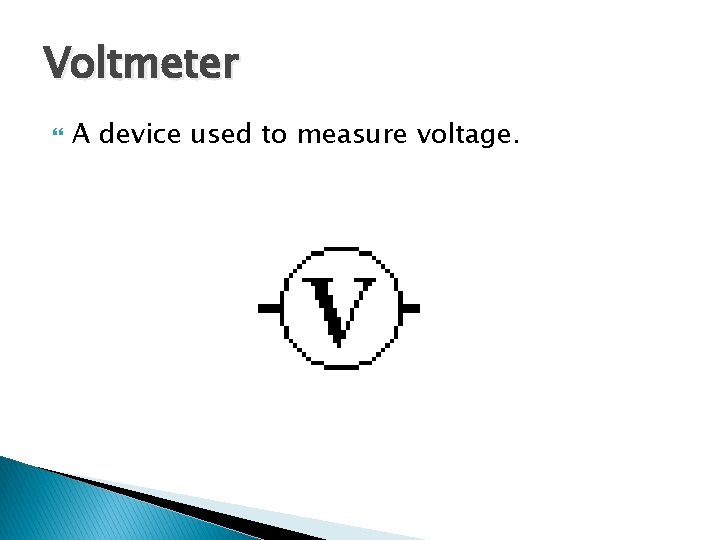Voltmeter A device used to measure voltage.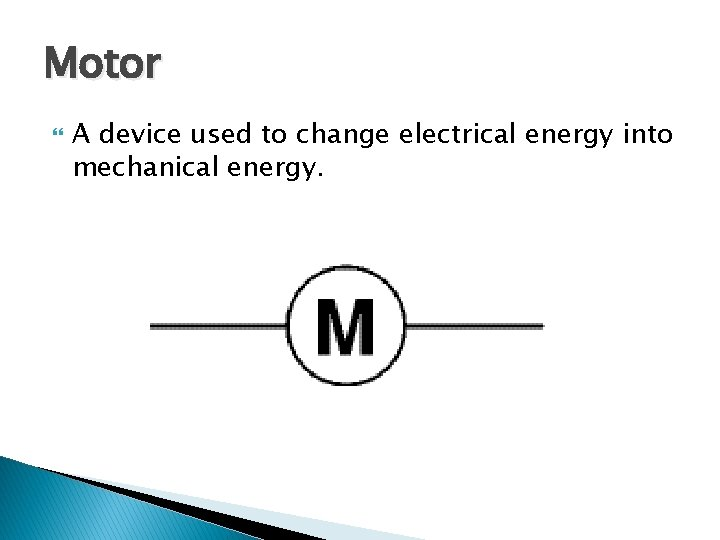Motor A device used to change electrical energy into mechanical energy.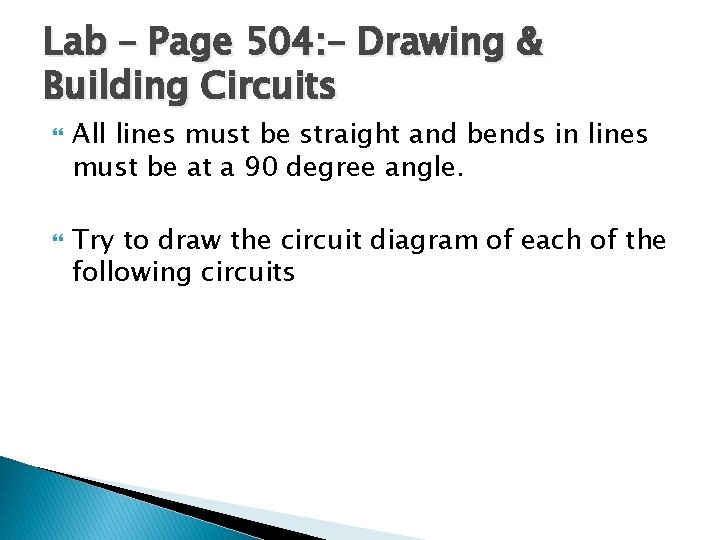Lab – Page 504: – Drawing & Building Circuits All lines must be straight and bends in lines must be at a 90 degree angle. Try to draw the circuit diagram of each of the following circuitsCircuit 1 Series circuitCircuit 2 Series circuit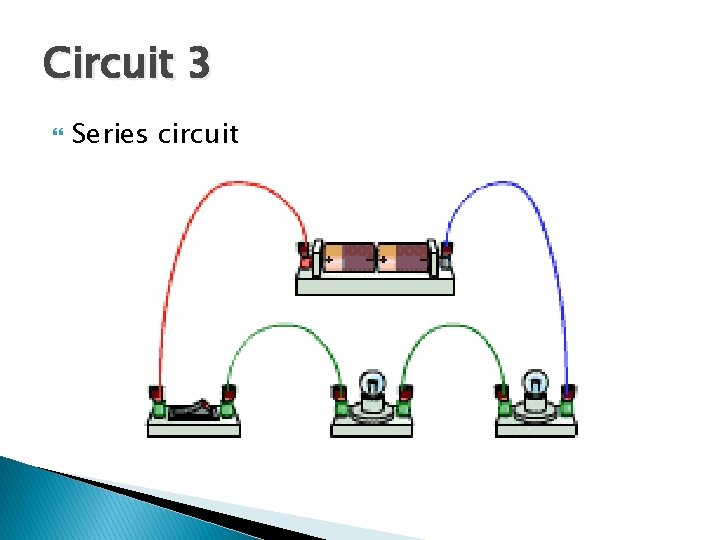Circuit 3 Series circuit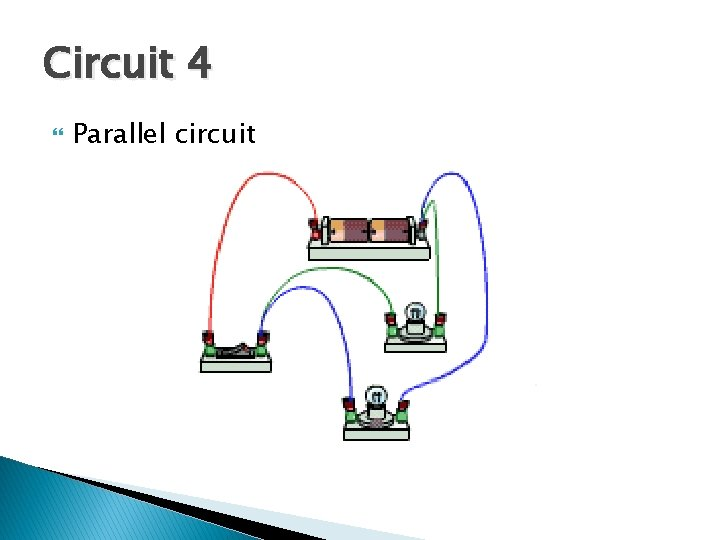Circuit 4 Parallel circuit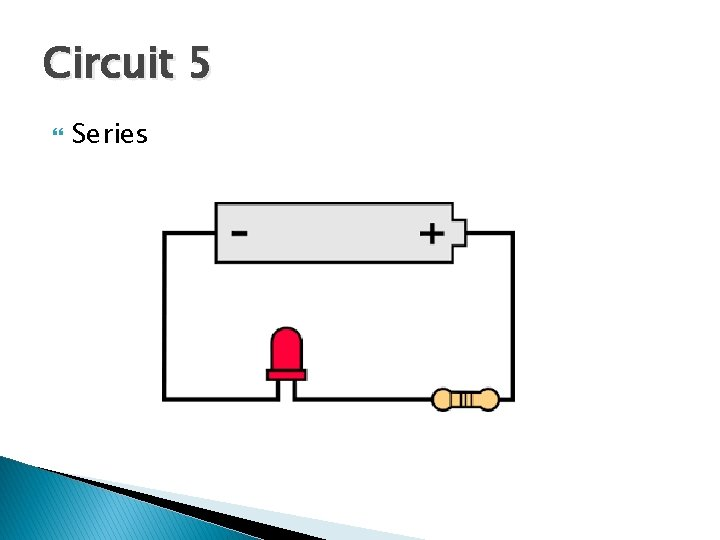Circuit 5 Series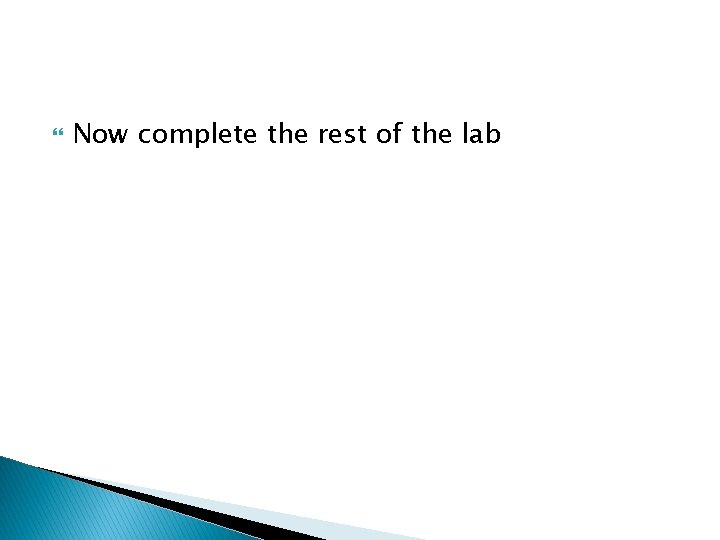Now complete the rest of the lab